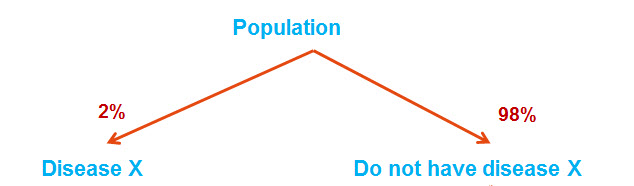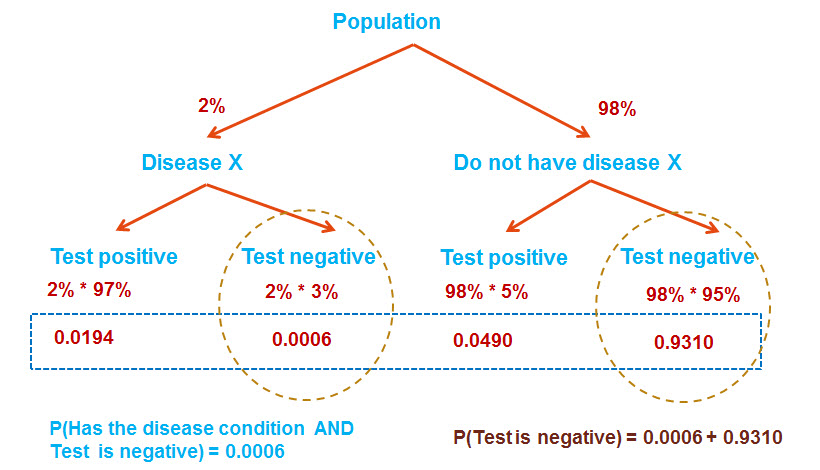Conditional probability problem 1

Question (Conditional probability problems): In a class 80% of students like coffee; 60% students like both tea and coffee. What percentage of students who like coffee also like tea?

Show Solution

Solution:

A – Like Coffee

B – Like Tea

P(A) = 0.8

P(A AND B) = 0.6

Required – Percentage of students who like coffee also like tea

This is same as finding out the probability that a student likes tea given that he/she likes coffee.

Required probability = P(B | A) = P(Like tea | Like Coffee) { Interpretation –  P( Like tea given that he likes coffee)}

P(B | A) = P(B AND A) / P(A)

= 0.6/0.8 = 0.75

75% of students who like coffee also like tea.

Conditional probability problem 2

Question (Conditional probability problems): In a class 40% of students play football.

It is known that probability that a randomly selected student who plays football  also plays baseball is 0.2. Probability that a randomly selected student who plays baseball also plays football is 0.8.

Find out the probability that a randomly selected student plays baseball?

Show Solution

Solution:

A – Plays football

B – Plays Baseball

Given information:

P(A) = 0.4

P(B | A) = 0.2  { Probability that a student plays Baseball given that he plays football}

P(A | B) = 0.8 { Probability that a student plays Football given that he plays Baseball}

Required probability = P(B)

From Bayes theorem

0.2 = 0.8 * P(B)/0.4

P(B) = 0.1  {probability that a randomly selected student plays baseball}

Conditional probability problem 3

Question (Conditional probability problems):

For a certain disease, probability that a person will test positive is 0.15.

The test’s accuracy has been a concern; 20% of those who test positive do not actually have the disease. On the other hand, 5% of those who tested negative for the test actually have the disease.

1. Given that a person has disease what is the probability that he will test negative?
2. Find the probability a randomly selected person will not have disease.
3. Probability that a person will test positive given that he/she does not have disease.

Show Solution

Solution:

For the given scenario, all the possibilities along with respective probabilities are depicted below.1. Required probability = P (Negative | Disease) = P ( Negative AND Disease) / P (Disease)

P (Negative AND Disease) = C

P (Disease) = A + C

Required probability = C / (A + C) = (0.85*0.05) / (0.15*0.8 + 0.85*0.05) = 0.26

1. P ( No Disease) = B + D = 0.15*0.2 + 0.85*0.95 = 0.8375

1. P (Positive | No Disease) = P (Positive AND No Disease) / P (No Disease)

= B / (B + D) = 0.15*0.2 / (0.8375) = 0.0358

Conditional probability problem 4

Question (Conditional probability problems): For events, A and B probabilities are given below

P(A | B) = 0.3

P(B | A) = 0.2

P(A U B) = 0.9

Are the events A and B independent?

Show Solution

Solution:

P(A | B) = P(A B) / P(B) = 0.3

Let P(A B) = x

P(B) = x/0.3 = 10x/3

P(B | A) = P(A B) / P(A) = 0.2

P(A) = x/0.2 = 5x

P(A U B) = P(A) + P(B)  –  P(A B)

= (5x) + (10x/3) – (x) = 0.9

x = 0.122

P(A B)  = 0.123

P(A)*P(B) =  0.252

If events A and B are independent then P(A ∩ B)  = P(A) * P(B)

Hence, A and B are not independent.

Conditional probability problem 5

Question (Conditional probability problems):

An urn contains 15 marbles (5 green and 10 red). If three marbles are drawn one after the other successively without replacement. What is the probability that the third marble drawn is red given that the first marble drawn is green?

Show Solution

Solution:

Tree diagram approach is useful in solving such problems.

Probability of green, blue and red marbles is as shown below.

P (G) = 5/15 = 1/3

P (R) = 10/15 = 2/3

Below tree diagram shows the list of all possibilities for drawing three marbles as well as the respective probabilities.Required probability = P (R | 1st green marble)

Below is the highlighted set of possibilities where the 1st marble drawn is greenAmong this set, we need to look for the third marble being red.

Highlighted in blue are the final possibilities where the third marble drawn is blue given that 1st marble is green.Required probability is sum of the highlighted probabilities

P =  (1/3 * 4/14 * 10/13) +  (1/3 * 10/14 * 9/13) = 0.238 ~ 0.24

Conditional probability problem 6

Question (Conditional probability problems): Three fair dice are thrown simultaneously. What is the probability that the numbers on first two dice are equal given that sum of numbers on all three dice is 14?

Show Solution

Solution:

Let us define the events involved

Event A – Sum of all three numbers is 15

Event B – Numbers are equal on first two dice

Required probability is P (B | A)

Total possibilities when 3 dice are rolled = 6*6*6 = 216

In total we have 15 possibilities for sum of numbers on all 3 dice = 14

{2, 6, 6}, {3, 5, 6}, {3, 6, 5}, {4, 4, 6}, {4, 5, 5}, {4, 6, 4}, {5, 3, 6}, {5, 4, 5},

{5, 5, 4}, {5, 6, 3}, {6, 2, 6}, {6, 3, 5}, {6, 4, 4}, {6, 5, 3}, {6, 6, 2}

Of these 15 possibilities, we have below 3 that have first two numbers equal.

{4, 4, 6}, {5, 5, 4}, {6, 6, 2}

P (B | A) = P (B AND A)/ P (A)

P (B AND A) = 3/216

P (A) = 15/216

P (B | A) = 3/15 = 0.2

Conditional probability problem 7

Question (Conditional probability problems): Team A has to win two more matches to reach ‘Finals’. Probability that it wins both the matches is 0.3 and probability that team ‘A’ wins second match is 0.4. Find the probability that team ‘A’ wins second match given that it already won first match.

Show Solution

Solution:

Let X and Y be the events that represent team ‘A’ winning first and second match respectively.

P(X AND Y) = 0.3

P(Y) = 0.4

Required probability = P(Y | X) = P(X AND Y) / P(X) = 0.3/0.4 = 0.75

Conditional probability problem 8

Question (Conditional probability problems):

An urn contains red and green marbles. Probability of drawing a green marble is 0.4. Probability of drawing a red marble given that a green marble is drawn is 0.2. If two marbles are drawn without replacement find the probability of drawing a red marble.

Show Solution

Solution:

As the urn contains only Red & Green marbles and also it is known the probability of drawing green marble is 0.4, probability of getting red marble is simply 1 – 0.4 = 0.6

Conditional probability problem 9

Question (Conditional probability problems):

Consider a city where probability that it rains on a given day is 0.06. Probability that traffic jams occur in this city is 0.14. Probability that there will be traffic jam on a rainy day is 0.8. Find the probability that it rains given that the city is experiencing traffic jam that day.

Show Solution

Solution:

P (Rains) = P(R) = 0.06

P (Traffic Jam) = P (T) = 0.14

P (T | R) = 0.8

P (T | R) = P (T AND R) / P (R)    (Equation 1)

Required probability = P (Rains | Traffic Jam) = P (R | T)

P (R | T) = P (R AND T) / P (T)

Replace P (R AND T) with P (T | R) * P (R)  (Using equation 1)

P (R | T) = P (T | R) * P (R) / P (T)

= 0.8 * 0.06/0.14 = 0.34

Conditional probability problem 10

Question (Conditional probability problems): It is known that the 2% of all the population in a country are affected by a particular disease condition ‘X’.

A certain diagnostic test for detecting the presence of this disease condition gives accurate results 97% of the times when the person actually have the disease condition and yields 95% accurate results when the person actually do not have the disease condition.

Given that a person tests negative for diagnostic test, what is the probability that the person actually has the disease?

Show Solution

Solution:

Such problems can be solved using the tree diagram approach. Tree diagram details all possible events along with the corresponding probabilities.

STEP 1:

Start with the entire population of the country

Two possibilities as shown belowSTEP 2:

Once the individual go for the diagnostic test, two possibilities for the test result (Positive or Negative) regardless of actual disease condition. Hence, each node has two branches again as shown below.Quick check – Check if all the possibilities are covered. If so, the sum of all probabilities at the lowest level is equal to 1.STEP 4:

Identify the required probability:Given that a person tests negative for diagnostic test, what is the probability that the person actually has the disease?

P(Has the disease condition | Test is negative) =

P(Has the disease condition  AND  Test is negative)/P(Test is negative)

As shown in the highlighted portion below,

P(Has the disease condition  AND  Test is negative) = 0.0006/ (0.0006 + 0.9310) = 0.000644